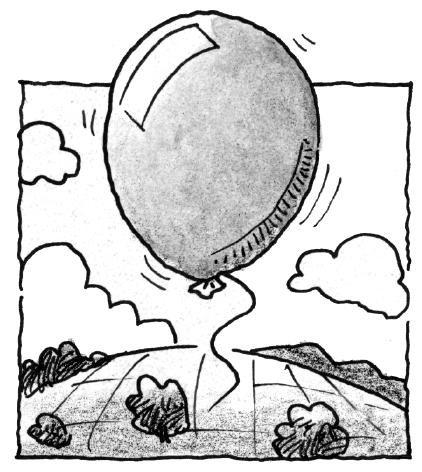### Home > APCALC > Chapter 2 > Lesson 2.3.2 > Problem2-119

2-119.

When the balloon in problem 2-81 reached $500$ feet, it popped and started to fall back towards the ground. The height of the balloon as it falls can be modeled by the function $h(t)=-16t^2+500$, where $t$ is the number of seconds since the time it popped and $h(t)$ is the height of the balloon (in feet) above the ground.

1. According to the model, when will the balloon hit the ground?

What value of $h$ represents the ground?

2. Approximate the balloon’s velocity at $t = 5$ seconds.

Velocity is slope of a distance/position graph. Since $h(t)$ is a parabola, we do not have the skills (yet) to find the slope on a curve. But we can approximate.

Choose two times very close to $t = 5$. Use the function, $h\left(t\right)$, to find the balloon's height at those times. Find the slope of the line that goes through those points. This will be a good approximation of the velocity of the balloon at $t = 5$.